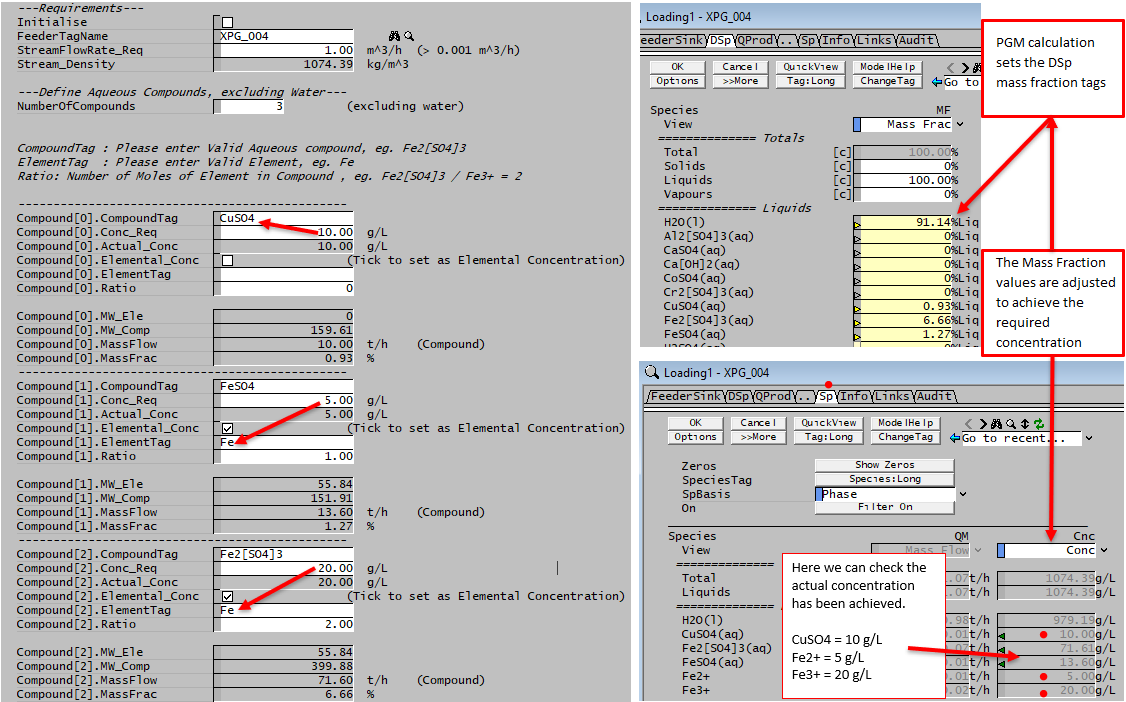# Aqueous Feed Concentration Calculator

Navigation: PGMs ➔ Example PGM Files ➔ Aqueous Feed Concentration Calculator

SysCAD Pre-Defined Classes and Examples EXAMPLES: User Defined Classes
Sp Databse
Class
PSD
Class
Array
Class
StrArray
Class
Matrix
Class
Tag Select
Class
Plant Model
Class
Noise
Class
Time
Class
Agitator
Power
Reaction
Finder
Aq Feed Conc
Calculator
Evaporation
Correlation
Bayer Liquor
Class
Check
Element Bal

Related Links: PGM Classes, PGM Functions

## PGM File - Aqueous Feed Concentration Calculator

```;--- SysCAD General Controller (PGM) file ---
; Revision: 2     Date:  18/Oct/2019    Author: KWA KENWALT
; This PGM will calculate the Feed composition (in a single feeder) based on user specified aqueous concentration.
; See example at the end of the page.

Class	CompoundDef
TextLabel("  -----------------------------------------------")
String	  CompoundTag*
REAL	  Conc_Req*("Conc", "g/L")
REAL	  [email protected]("Conc", "g/L")
Checkbox  Elemental_Conc*{Comment("Tick to set as Elemental Concentration")}
String	  ElementTag*
REAL	  Ratio*
TextLabel()
REAL	  [email protected]@, [email protected]@
REAL	  [email protected]@("Qm", "t/h"){Comment("Compound")}
REAL	  [email protected]@("Frac", "%")

Sub exec()
MW_Ele = IIF(Elemental_Conc, [Concatenate("\$SDB.Elem.", ElementTag, ".MoleWt")], 0)
MW_Comp = [Concatenate("\$SDB.", CompoundTag, "(aq).MoleWt()")]
MassFlow = IIF(Elemental_Conc, (Conc_Req * MW_Comp / (MW_Ele*Ratio)), Conc_Req)
EndSub

Sub Hide()
SetConcealedState(this, True)
EndSub

Sub Show()
SetConcealedState(this, False)
EndSub
EndClass

PageLabel("Inputs")
TextLabel(, "  ---Requirements---")
Checkbox  Initialise*
String	  FeederTagName*{Tag}
REAL	  StreamFlowRate_Req*("Qv", "m^3/h")<0.001,>
;TextLabel(, "---Basis MassFlow---")
REAL	  Guess_Density**("Rho", "kg/m^3"){Comment("Initial Guess")}<1000,>
REAL	  [email protected]("Rho", "kg/m^3"), [email protected]@("Rho", "kg/m^3")
REAL	  Basis_MassFlow**("Qm", "t/h"){Comment("Basis used to workout Mass fraction")}<1000,>
REAL	  [email protected]@("Qm", "t/h")
REAL	  [email protected]@("Qm", "t/h"){Comment("by difference")}
REAL	  [email protected]@("Qm", "t/h")
REAL	  [email protected]@("Frac", "%"){Comment("by difference")}

TextLabel(,"  ---Define Aqueous Compounds, excluding Water---")
Byte	  NumberOfCompounds*{Comment("excluding water")}
TextLabel(,)
TextLabel("  CompoundTag : Please enter Valid Aqueous compound, eg. Fe2[SO4]3 ")
TextLabel("  ElementTag  : Please enter Valid Element, eg. Fe ")
TextLabel("  Ratio: Number of Moles of Element in Compound , eg. Fe2[SO4]3 / Fe3+ = 2 ",)

CompoundDef Compound

Byte	  i, j, k, n
String	  ReqdSpecie
long	  NumSpecies, LiqPhaseIndex, SpeciePhase, x

Sub InitialiseSolution()
;--- Set Stream Flowrate, assume volumetric flowrate is used.
Initialise = 1

;--- Preset all aqueous species to 0%, water to 100%
NumSpecies = SDB.SpecieCount()
LiqPhaseIndex = SDB.FindPhase("l")
x = 0
while (x < Numspecies)
SpeciePhase = SDB.SpPhaseNo(x)
if (SpeciePhase == LiqPhaseIndex)
ReqdSpecie = SDB.SpSymbol(x)
[Concatenate(FeederTagName,".Content.MF:Ph.", ReqdSpecie ," (%Liq)")] = 0
endif
x = x + 1
endwhile

[Concatenate(FeederTagName,".Content.MF:Ph.H2O(l) (%Liq)")] =  100

;--- Show the required compound tags
n = 0
While n < 20
if (n<=NumberOfCompounds)
Compound[n].Show()
endif
n = n + 1
EndWhile

;--- Initialise Feedrate if no feed has been defined, a value is needed to calculate concentration
[Concatenate(FeederTagName,".QvReqd (m^3/h)")] = StreamFlowRate_Req

;---- Initialise Density
If (Stream_Density < Guess_Density)
Stream_Density = Guess_Density
Endif
EndSub

Sub TerminateSolution()
;--- Gets the actual concentration when the project is stopped, Gets the Concentration of Compound or Element, based on the defined method.
j= 0
While j < NumberOfCompounds
Compound[j].Actual_Conc = [Concatenate(FeederTagName,".QProd.Cnc:Ph.",Compound[j].CompoundTag, "(aq) (g/L)")]
Compound[j].Actual_Conc = IIF(Compound[j].Elemental_Conc, Compound[j].Actual_Conc / Compound[j].MW_Comp * (Compound[j].MW_Ele * Compound[j].Ratio), Compound[j].Actual_Conc)
j= j + 1
EndWhile
n = 0
While n < 20
if (n>=NumberOfCompounds)
Compound[n].Hide()
endif
n = n + 1
EndWhile
EndSub

;--- This logic executed at EVERY step ---

If Stream_Density <> NewDensity  OR NewDensity < 0.1  OR Initialise
Stream_Density = NewDensity

;Calculates the mass flow of non water aqueous species based on current density
i = 0
Total_NonWaterFlow = 0
While i < NumberOfCompounds
Compound[i].Exec()
Total_NonWaterFlow = Total_NonWaterFlow + Compound[i].MassFlow
i = i + 1
Endwhile

;Calculates the mass flow of water based on current density
H2O_MassFlow    = Basis_MassFlow * (Stream_Density/Basis_MassFlow) - Total_NonWaterFlow
Total_BasisFlow = H2O_MassFlow + Total_NonWaterFlow

;Calculates	and sets the mass fraction of the aqueous species
k = 0
While k < NumberOfCompounds
Compound[k].MassFrac 	= Compound[k].MassFlow / Total_BasisFlow * 100
[Concatenate(FeederTagName,".Content.MF:Ph.",Compound[k].CompoundTag,"(aq) (%Liq)")] = Compound[k].MassFrac
k = k + 1
EndWhile

H2O_MassFrac = H2O_MassFlow / Total_BasisFlow * 100
[Concatenate(FeederTagName,".Content.MF:Ph.H2O(l) (%Liq)")] = H2O_MassFrac

Initialise = 0

Endif

NewDensity = [Concatenate(FeederTagName,".QProd.Rho (kg/m^3)")]

\$ ; --- end of file ---
```

## Using the Aqueous Feed Concentration Calculator in a Project

Steps Example
1. Add a General Controller in the project and Load in the pgm file above.
2. Fill in the Feeder Tag Name
3. Specify a Stream Flowrate (must have a flow to complete the calculations)
4. Specify the Number of aqueous compounds to be defined (excluding water)
5. Fill in the compound information (per compound)
• Define the compound, must be a valid compound in the project in the (aq) form.
• Define the required concentration in g/L @ Temperature
• If elemental concentration is used, tick the Elemental_Conc checkbox and defined the Element Tag as well as the molar ratio (compound : Element)
6. When all the data is filled in, press OK to save the changes
7. Run the project. SysCAD will adjust the composition in the nominated Feeder to meet the required concentration.

Notes:

• The number of converged iterations within the project may need to be increased for this controller to achieve its setpoints in the downstream pipe.
• In this example PGM, we have allowed up to 20 aqueous compounds, we have used the subroutine "Hide" to conceal any unused blank input fields. If you want to run the aqueous calculation again by adding more compounds, you may have to press the "Show Concealed" button the on "GControl" tab first to show the concealed fields. Alternatively, you can solve the project for at least 1 iteration to get the PGM to unhide the concealed input fields.